## Mixture density networks

### Conditional Probability

Conditional probability is the probability of one event occurring with some relationship to one or more other events. For example:

Event A is that it is raining outside, and it has a 0.3 (30%) chance of raining today. Event B is that you will need to go outside, and that has a probability of 0.5 (50%). A conditional probability would look at these two events in relationship with one another, such as the probability that it is both raining and you will need to go outside.

The formula for conditional probability is: P(B|A) = P(A and B) / P(A) which you can also rewrite as: P(B|A) = P(A∩B) / P(A)

-> 0.3*0.5 / 0.3 = 0.5 (50%)

### How do Mixture Density RNNs Predict the Future?

#### https://arxiv.org/pdf/1901.07859.pdf

In practice, a mixture density network (MDN) operates by transforming the outputs of a neural network to form the parameters of a mixture distribution (Bishop, 1994), generally

with Gaussian models for each mixture component. These parameters are the centres (µ) and scales (σ) for each Gaussian component, as well as a weight (π) for each component (see Figure 1). The MDN usually uses an exponential activation function to transform the scale parameters to be positive and non-zero. For training, the probability density function of the mixture model is used to generate the negative log likelihood for the loss function. This involves constructing probability density functions (PDFs) for each Gaussian component and categorical distribution from the mixture weights (see Appendix Section 1.4 for details). One advantage of an MDN is that various component distributions can be used so long as the PDF is tractable, for instance, 1D (Bishop, 1994) or 2D (Graves, 2013) Gaussian distributions, or, as in our case, a multivariate Gaussian with a diagonal covariance matrix. For inference, results are sampled from the mixture distribution. First, the πs are used to form a categorical distribution by applying the softmax function. A sample is drawn from this distribution to determine which Gaussian component will provide the output. The index i of the sampled π is used to select a Gaussian distribution, N (µi , σ2 i ), from which a sample is drawn to provide the outcome. In some cases, it is advantageous to adjust the diversity of sampling (for instance, to favour unlikely predictions), in which case the temperature of the categorical distribution can be adjusted in the typical way, and the covariance matrices of the Gaussian components may be scaled. We refer the these operations as adjusting π- or σ-temperature respectively.

In :
``````import matplotlib.pyplot as plt
import numpy as np
import tensorflow as tf
import math``````
In [ ]:
`` ``
In :
``````NSAMPLE = 1000
x_data = np.float32(np.random.uniform(-10, 10, (1, NSAMPLE))).T
r_data = np.float32(np.random.normal(size=(NSAMPLE,1)))
y_data = np.float32(np.sin(0.75*x_data)*7.0+x_data*0.5+r_data*1.0)

plt.figure(figsize=(8, 8))
plot_out = plt.plot(x_data,y_data,'ro',alpha=0.3)
plt.show()
``````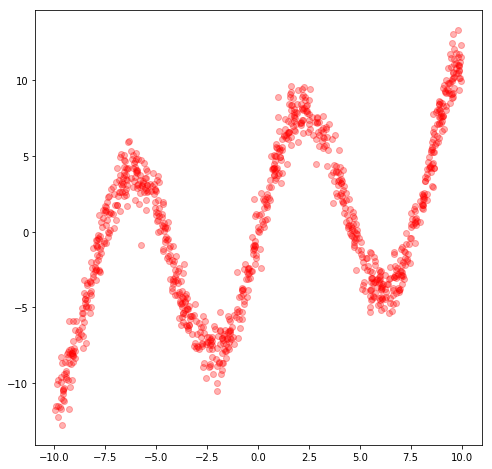In :
``````x = tf.placeholder(dtype=tf.float32, shape=[None,1])
y = tf.placeholder(dtype=tf.float32, shape=[None,1])

``````
In :
``````#####
NHIDDEN = 20
W = tf.Variable(tf.random_normal([1,NHIDDEN], stddev=1.0, dtype=tf.float32))
b = tf.Variable(tf.random_normal([1,NHIDDEN], stddev=1.0, dtype=tf.float32))

W_out = tf.Variable(tf.random_normal([NHIDDEN,1], stddev=1.0, dtype=tf.float32))
b_out = tf.Variable(tf.random_normal([1,1], stddev=1.0, dtype=tf.float32))

hidden_layer = tf.nn.tanh(tf.matmul(x, W) + b)
y_out = tf.matmul(hidden_layer,W_out) + b_out
``````
```WARNING:tensorflow:From /usr/local/lib/python3.6/dist-packages/tensorflow/python/framework/op_def_library.py:263: colocate_with (from tensorflow.python.framework.ops) is deprecated and will be removed in a future version. Instructions for updating: Colocations handled automatically by placer. ```
In :
``lossfunc = tf.nn.l2_loss(y_out-y);``
In :
``train_op = tf.train.RMSPropOptimizer(learning_rate=0.1, decay=0.8).minimize(lossfunc)``
In :
``````sess = tf.InteractiveSession()
sess.run(tf.initialize_all_variables())
``````
In :
``````NEPOCH = 5000
for i in range(NEPOCH):
sess.run(train_op,feed_dict={x: x_data, y: y_data})

``````
In :
``````x_test = np.float32(np.arange(-10,10,0.1))
x_test = x_test.reshape(x_test.size,1)
y_test = sess.run(y_out,feed_dict={x: x_test})

plt.figure(figsize=(8, 8))
plt.plot(x_data,y_data,'ro', x_test,y_test,'bo',alpha=0.3)
plt.show()

sess.close()
``````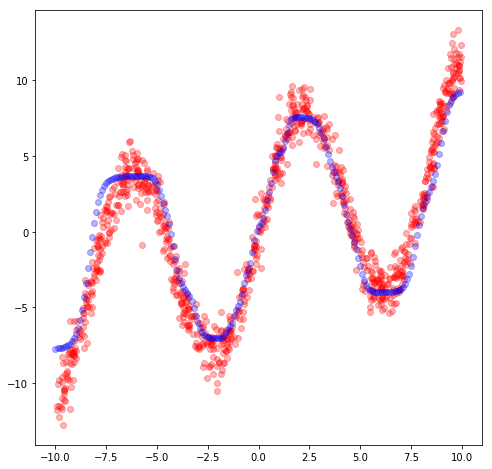In :
``````temp_data = x_data
x_data = y_data
y_data = temp_data

plt.figure(figsize=(8, 8))
plot_out = plt.plot(x_data,y_data,'ro',alpha=0.3)
plt.show()
``````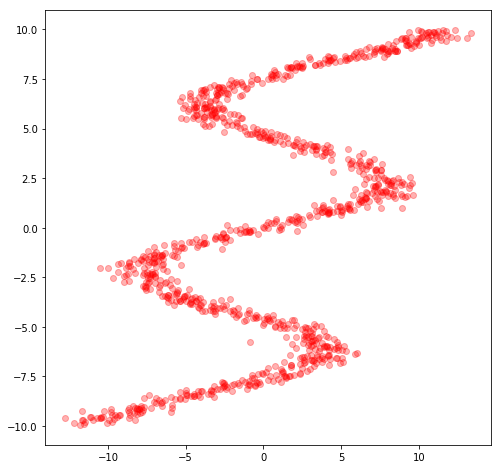In :
``````sess = tf.InteractiveSession()
sess.run(tf.initialize_all_variables())

for i in range(NEPOCH):
sess.run(train_op,feed_dict={x: x_data, y: y_data})

x_test = np.float32(np.arange(-10,10,0.1))
x_test = x_test.reshape(x_test.size,1)
y_test = sess.run(y_out,feed_dict={x: x_test})

plt.figure(figsize=(8, 8))
plt.plot(x_data,y_data,'ro', x_test,y_test,'bo',alpha=0.3)
plt.show()

sess.close()
``````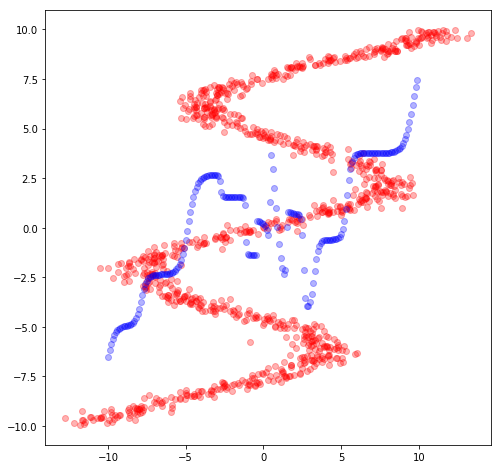In :
``````NHIDDEN = 24
STDEV = 0.5
KMIX = 24 # number of mixtures
NOUT = KMIX * 3 # pi, mu, stdev

x = tf.placeholder(dtype=tf.float32, shape=[None,1], name="x")
y = tf.placeholder(dtype=tf.float32, shape=[None,1], name="y")

Wh = tf.Variable(tf.random_normal([1,NHIDDEN], stddev=STDEV, dtype=tf.float32))
bh = tf.Variable(tf.random_normal([1,NHIDDEN], stddev=STDEV, dtype=tf.float32))

Wo = tf.Variable(tf.random_normal([NHIDDEN,NOUT], stddev=STDEV, dtype=tf.float32))
bo = tf.Variable(tf.random_normal([1,NOUT], stddev=STDEV, dtype=tf.float32))

hidden_layer = tf.nn.tanh(tf.matmul(x, Wh) + bh)
output = tf.matmul(hidden_layer,Wo) + bo

def get_mixture_coef(output):
out_pi = tf.placeholder(dtype=tf.float32, shape=[None,KMIX], name="mixparam")
out_sigma = tf.placeholder(dtype=tf.float32, shape=[None,KMIX], name="mixparam")
out_mu = tf.placeholder(dtype=tf.float32, shape=[None,KMIX], name="mixparam")

out_pi, out_sigma, out_mu = tf.split(output,3,1)

max_pi = tf.reduce_max(out_pi, 1, keepdims=True)
out_pi = tf.subtract(out_pi, max_pi)

out_pi = tf.exp(out_pi)

normalize_pi = tf.reciprocal(tf.reduce_sum(out_pi, 1, keep_dims=True))
out_pi = tf.multiply(normalize_pi, out_pi)

out_sigma = tf.exp(out_sigma)

return out_pi, out_sigma, out_mu

out_pi, out_sigma, out_mu = get_mixture_coef(output)

``````
```WARNING:tensorflow:From <ipython-input-12-6ab90a0555f6>:30: calling reduce_sum_v1 (from tensorflow.python.ops.math_ops) with keep_dims is deprecated and will be removed in a future version. Instructions for updating: keep_dims is deprecated, use keepdims instead ```
In :
``````NSAMPLE = 2500

y_data = np.float32(np.random.uniform(-10.5, 10.5, (1, NSAMPLE))).T
r_data = np.float32(np.random.normal(size=(NSAMPLE,1))) # random noise
x_data = np.float32(np.sin(0.75*y_data)*7.0+y_data*0.5+r_data*1.0)

plt.figure(figsize=(8, 8))
plt.plot(x_data,y_data,'ro', alpha=0.3)
plt.show()
``````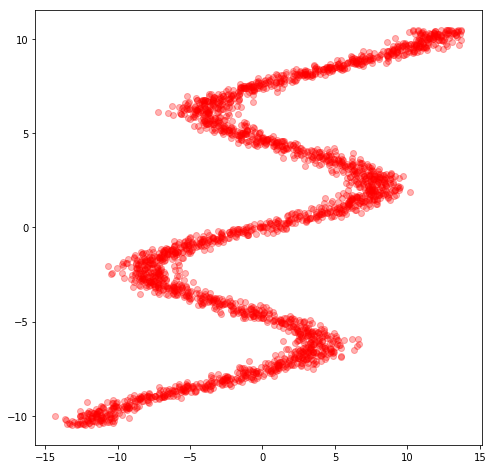\(((y-mu)*(1/sigma)) * (1/pi^2) \)

In [ ]:
`` ``
In :
``````oneDivSqrtTwoPI = 1 / math.sqrt(2*math.pi) # normalisation factor for gaussian, not needed.
def tf_normal(y, mu, sigma):
result = tf.subtract(y, mu)
result = tf.multiply(result,tf.reciprocal(sigma))
result = -tf.square(result)/2
return tf.multiply(tf.exp(result),tf.reciprocal(sigma))*oneDivSqrtTwoPI

def get_lossfunc(out_pi, out_sigma, out_mu, y):
result = tf_normal(y, out_mu, out_sigma)
result = tf.multiply(result, out_pi)
result = tf.reduce_sum(result, 1, keep_dims=True)
result = -tf.log(result)
return tf.reduce_mean(result)

lossfunc = get_lossfunc(out_pi, out_sigma, out_mu, y)
train_op = tf.train.AdamOptimizer().minimize(lossfunc)
``````
```WARNING:tensorflow:From /usr/local/lib/python3.6/dist-packages/tensorflow/python/ops/math_ops.py:3066: to_int32 (from tensorflow.python.ops.math_ops) is deprecated and will be removed in a future version. Instructions for updating: Use tf.cast instead. WARNING:tensorflow:From /usr/local/lib/python3.6/dist-packages/tensorflow/python/ops/math_grad.py:102: div (from tensorflow.python.ops.math_ops) is deprecated and will be removed in a future version. Instructions for updating: Deprecated in favor of operator or tf.math.divide. ```
In :
``````sess = tf.InteractiveSession()
sess.run(tf.initialize_all_variables())

NEPOCH = 10000
loss = np.zeros(NEPOCH) # store the training progress here.
for i in range(NEPOCH):
sess.run(train_op,feed_dict={x: x_data, y: y_data})
loss[i] = sess.run(lossfunc, feed_dict={x: x_data, y: y_data})
``````
In :
``````plt.figure(figsize=(8, 8))
plt.plot(np.arange(100, NEPOCH,1), loss[100:], 'r-')
plt.show()
``````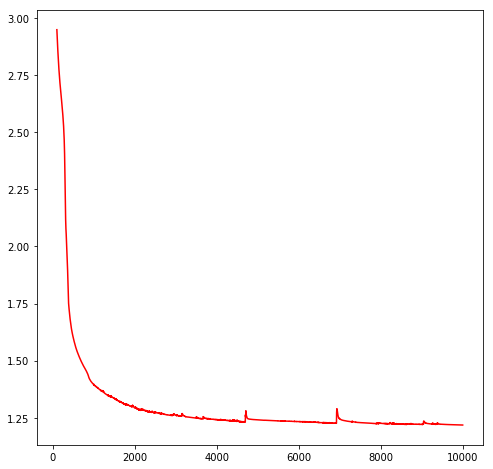In :
``````x_test = np.float32(np.arange(-15,15,0.1))
NTEST = x_test.size
x_test = x_test.reshape(NTEST,1) # needs to be a matrix, not a vector

def get_pi_idx(x, pdf):
N = pdf.size
accumulate = 0
for i in range(0, N):
accumulate += pdf[i]
if (accumulate >= x):
return i
print('error with sampling ensemble')
return -1

def generate_ensemble(out_pi, out_mu, out_sigma, M = 10):
NTEST = x_test.size
result = np.random.rand(NTEST, M) # initially random [0, 1]
rn = np.random.randn(NTEST, M) # normal random matrix (0.0, 1.0)
mu = 0
std = 0
idx = 0

# transforms result into random ensembles
for j in range(0, M):
for i in range(0, NTEST):
idx = get_pi_idx(result[i, j], out_pi[i])
mu = out_mu[i, idx]
std = out_sigma[i, idx]
result[i, j] = mu + rn[i, j]*std
return result``````
In :
``````out_pi_test, out_sigma_test, out_mu_test = sess.run(get_mixture_coef(output), feed_dict={x: x_test})

y_test = generate_ensemble(out_pi_test, out_mu_test, out_sigma_test)

plt.figure(figsize=(8, 8))
plt.plot(x_data,y_data,'ro', x_test,y_test,'bo',alpha=0.3)
plt.show()

``````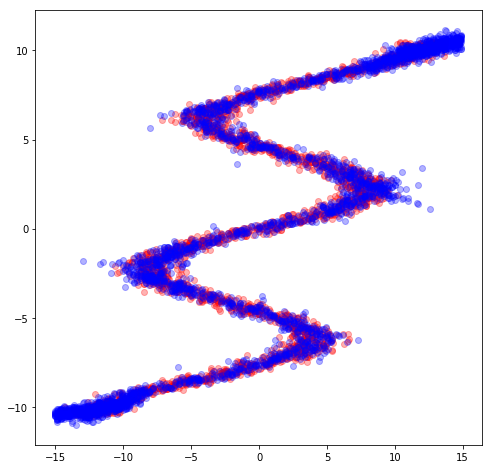In :
``````
sess = tf.InteractiveSession()
sess.run(tf.initialize_all_variables())

x = tf.constant([[1, 1, 1], [1, 2, 1]])
val = tf.constant(5.0)
op = tf.reduce_max(x,1)  # 6
rec = tf.reciprocal(val)
tf.reduce_sum(x, 0)  # [2, 2, 2]
tf.reduce_sum(x, 1)  # [3, 3]
tf.reduce_sum(x, 1, keepdims=True)  # [, ]
tf.reduce_sum(x, [0, 1])  # 6
val = sess.run(op)
print(val)
sess.close()
``````
```[1 2] ```